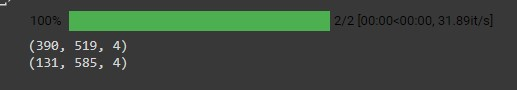# Tqdm bar is not changing

Hello, I’m trying to create progress bar using tqdm package.
Here is my code

``````import numpy as np
import os
from glob import glob
from PIL import Image
from tqdm.notebook import tqdm

path = glob('dataset/mnist_png/training/*/*.png')

height = []
width = []

for i in tqdm(path):
img_pil = Image.open(path)
image = np.array(img_pil)
h, w = image.shape
height.append(h)
width.append(w)

``````

However, progress bar does not changing and stops like this, even though the calculation is done
0%| | 0/60000 [00:00<?, ?it/s]

Is there any way to make progress bar changing?
I am looking forward to see any help. Thanks in advance.
Kind regards,
Yoon Ho

Already, your code seems to have a problem : you still use path in the loop

``````
for p in tqdm(path):

#img_pil = Image.open(path)

img_pil = Image.open(p)

# ...

``````

The code works for me without any problem.

``````
import numpy as np

import os

from glob import glob

from PIL import Image

#from tqdm import tqdm

from tqdm.notebook import tqdm

path = glob('/content/*.png')

height = []

width = []

print("")

for p in tqdm(path):

img_pil = Image.open(p)

image = np.array(img_pil)

print(image.shape)

h, w, _ = image.shape

height.append(h)

width.append(w)

``````